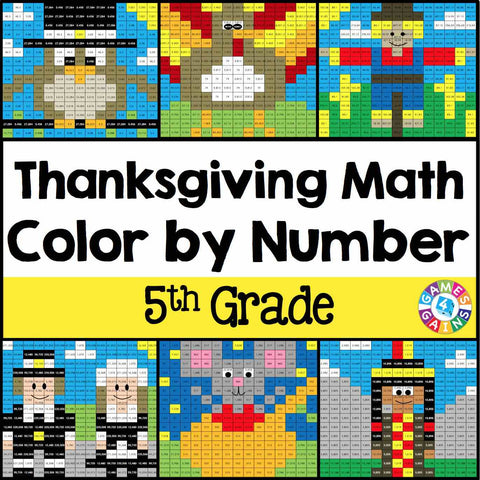Thanksgiving Math Color-by-Number - 5th Grade

• \$480

Thanksgiving Math Color-by-Number set comes with 6 Thanksgiving math color-by-number activities for reviewing 5th grade math skills. This Thanksgiving math set is perfect to use in centers, in small groups, or with the whole class!

Included with this Thanksgiving Math Color-by-Number set:
Instructions
6 color by number worksheets
6 student problem worksheets
6 teacher answer sheets

Here are the skills covered by these Thanksgiving math color-by-number activities:

• Multiplying and dividing decimals by 10, 100, and 1,000 (with the decimals in the problem written in standard, word, and expanded form)
• Ordering decimals
• Subtracting decimals from whole numbers
• Multiplying whole numbers (up to 3-digit x 3-digit and 4-digit x 2-digit)
• Solving multi-step word problems (whole numbers with addition, subtraction, and multiplication)
• Evaluating expressions with parentheses (whole numbers with addition, subtraction, and multiplication)

Want to learn more about what you'll get? Check out the preview to see a sample of the actual pages.